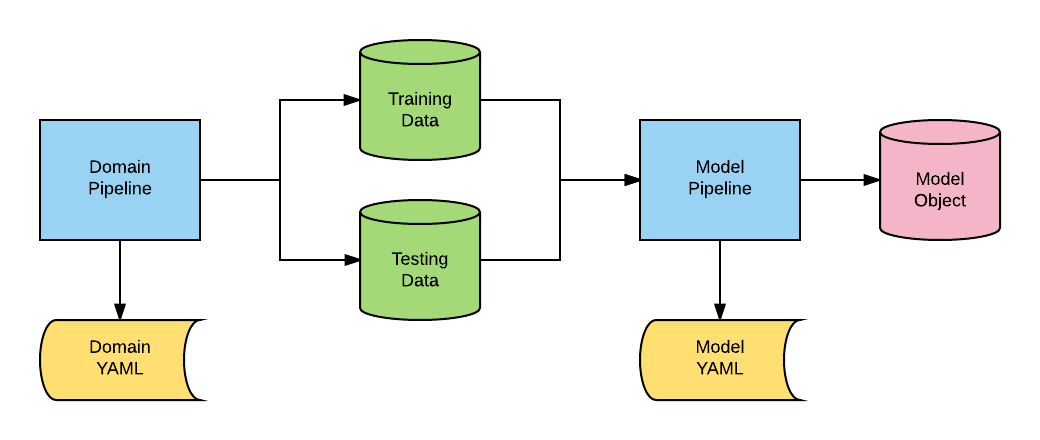# Introduction

AlphaPy is a machine learning framework for both speculators and data scientists. It is written in Python with the `scikit-learn` and `pandas` libraries, as well as many other helpful libraries for feature engineering and visualization. Here are just some of the things you can do with AlphaPy:

• Run machine learning models using `scikit-learn` and `xgboost`.

• Create models for analyzing the markets with MarketFlow.

• Predict sporting events with SportFlow.

• Develop trading systems and analyze portfolios using MarketFlow and Quantopian’s `pyfolio`.

The `alphapy` package is the base platform. The domain pipelines MarketFlow (`mflow`) and SportFlow (`sflow`) run on top of `alphapy`. As shown in the diagram below, we separate the domain pipeline from the model pipeline. The main job of a domain pipeline is to transform the raw application data into canonical form, i.e., a training set and a testing set. The model pipeline is flexible enough to handle any project and evolved over many Kaggle competitions.Let’s review all of the components in the diagram:

`Domain Pipeline`:

This is the Python code that creates the standard training and testing data. For example, you may be combining different data frames or collecting time series data from an external feed. These data are transformed for input into the model pipeline.

`Domain YAML`:

AlphaPy uses configuration files written in YAML to give the data scientist maximum flexibility. Typically, you will have a standard YAML template for each domain or application.

`Training Data`:

The training data is an external file that is read as a pandas dataframe. For classification, one of the columns will represent the target or dependent variable.

`Testing Data`:

The testing data is an external file that is read as a pandas dataframe. For classification, the labels may or may not be included.

`Model Pipeline`:

This Python code is generic for running all classification or regression models. The pipeline begins with data and ends with a model object for new predictions.

`Model YAML`:

The configuration file has specific sections for running the model pipeline. Every aspect of creating a model is controlled through this file.

`Model Object`:

All models are saved to disk. You can load and run your trained model on new data in scoring mode.

## Core Functionality

AlphaPy has been developed primarily for supervised learning tasks. You can generate models for any classification or regression problem.

• Binary Classification: classify elements into one of two groups

• Multiclass Classification: classify elements into multiple categories

• Regression: predict real values based on derived coefficients

Classification Algorithms:

• Extra Trees

• K-Nearest Neighbors

• Logistic Regression

• Support Vector Machine (including Linear)

• Naive Bayes (including Multinomial)

• Random Forests

• XGBoost Binary and Multiclass

Regression Algorithms:

• Extra Trees